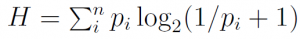# Hick’s Law

In the 50s William Hick (a British psychologist) examined the speed of perception and information processing. In his experiments he created tasks where the subjects had to select a specific information from a list of information. He measured the change of the time needed for a selection, when the list of information was reduced in size. . The evaluations of the experiments resulted in a logarithmic curve. He approximated the curve with the following formula for the rate of gain of information:

Formula in MathML format (not all browsers support this by now):

$H=∑inpilog2(1pi+1)$

Formula in PNG image format:The entrophy H is derived from the sum of the probabilities (pi) of possible selections multiplied by a logarithmic value (where n is the number of choices). The logarithm of this suggests that human beings do not perform a linear search in lists.  We rather try to divide them into categories. This allows us to exclude half the possibilities at each step.

Remark:
In HCI Hick’s law is used to compare different menu layouts in graphical user interfaces in order to determine which one is most effective.

: William Edmund Hick. On the rate of gain of information. Quarterly Journal of Experimental Psychology, 4(1):1126, 1952.